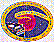### Sounds of solar oscillations (in MP3 format)

• one mode (l=1,n=20, nu=2.94-3.0 mHz), MP3 format, 8-bit, 11.025 kHz sampling rate (0.5 MB)
• three modes (l=0,n=21, l=1,n=20, l=2,n=20, nu=2.95-3.05 mHz), MP3 format, 8-bit, 11.025 kHz sampling rate (0.5 MB)
• all low-degree modes (l=0,1,2, and 3), MP3 format, 8-bit, 11.025 kHz sampling rate (0.5 MB)
• MP3 format, 16-bit, 44.1 kHz sampling rate, 22 min, sounds of the Sun for a whole year (10/1996-10/1997) (22 MB)
• ### Sounds of solar oscillations (in AIFF format)

• one mode (l=1,n=20, nu=2.94-3.0 mHz), AIFF format, 8-bit, 11.025 kHz sampling rate (0.9 MB)
• three modes (l=0,n=21, l=1,n=20, l=2,n=20, nu=2.95-3.05 mHz), AIFF format, 8-bit, 11.025 kHz sampling rate (0.9 MB)
• all low-degree modes (l=0,1,2, and 3), AIFF format, 8-bit, 11.025 kHz sampling rate (0.9 MB)

#### The sounds are obtained from 40-days of MDI disk-averaged data speeded up by factor 42,000.The SOI Main Page.